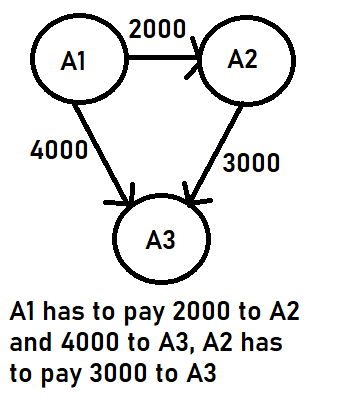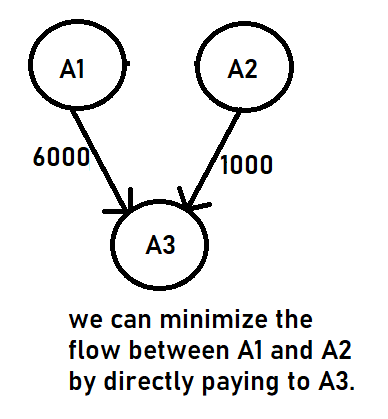# Minimize Cash Flow among a given set of friends who have borrowed money from each other

## Introduction

This problem is asked in one of the top companies like Amazon. If you are aiming for such companies, then this problem is a must.

What do you mean by minimizing cash flow?

Let's understand this with the help of the problem statement.

## Problem Statement

Suppose you are given that there are few friends. They have borrowed money from each other due to which there will be some cash flow on the network. Our main aim is to design an algorithm by which the total cash flow among all the friends is minimized.

For example:

There are three friends A1, A2, A3; you have to show the settlement of input debts between them.Note: Please try to solve the Minimizing Cash Flow on CodeStudio before stepping into the solution.

## Approach 1: Greedy Algorithm

The approach we will be using for minimizing cash flow is the Greedy Algorithm. The greedy approach is used to build the solution in pieces, and this is what we want to minimize the cash flow. At every step, we will settle all the amounts of one person and recur for the remaining n-1 persons.

Calculate the net amount for every person, which can be calculated by subtracting all the debts, i.e., the amount to be paid from all credit, i.e., the amount to be paid to him. After this, we will find two persons with the maximum and the minimum net amounts. The person with a minimum of two is our first person to be settled and removed from the list.

Following algorithm will be done for every person varying 'i' from 0 to n-1.

1. The first step will be to calculate the net amount for every person and store it in an amount array.
1. Net amount = sum(received money) - sum(sent money).
2. Find the two people that have the most credit and the most debt. Let the maximum amount to be credited from maximum creditor be max_credit and the maximum amount to be debited from maximum debtor be max_debit. Let the maximum debtor be debt and maximum creditor be cred.
3. Let the minimum of two amounts be 'y'.
4. If y equals max_credit, delete cred from the list and repeat for the remaining (n-1) people.
5. If y equals max_debit, delete debt from the group of people and repeat for recursion.
6. If the amount is 0, then the settlement is done.

Output

## Complexity Analysis

• Time Complexity: The time complexity of minimizing the cash flow is O(n^2). Where n = number of persons.
• Space Complexity:

1. What do you mean by minimizing cash flow?
Here minimizing cash flow means how efficiently or conveniently you can make a flow of cash transfer among your friends.

2. What do you mean by Greedy Algorithm?
Greedy is an algorithmic approach that assembles a solution piece by piece, constantly opting for the next piece that provides the most evident and immediate benefit. Greedy is best suited to problems when picking locally optimal leads to a global solution.

3. What is the time complexity of this approach?
The time complexity of this approach efficiently is O(n^2), where n is equal to the number of persons.

## Key Takeaways

In this blog, we discuss the problem's solution, where we have to minimize the cash flow among the friends.

We see the implementation of minimizing cash flow problems in the Java language. Apart from this, you can practice more questions similar to this problem or on a Greedy algorithm like Find minimum time to finish all jobs with given constraints,  Find maximum sum possible equal to the sum of three stacks, and many more.

You can also have a view of the Data Structures and Algorithms guided path to start your preparation from scratch.